The Boolean equation of NOR gate is
(a) C = A + B               (b) $\mathrm{C}=\overline{\mathrm{A}+\mathrm{B}}$
(c) $C=A·B$                 (d) $\mathrm{C}=\overline{\mathrm{A}.\mathrm{B}}$

Concept Questions :-

Logic gates
High Yielding Test Series + Question Bank - NEET 2020

Difficulty Level:

This symbol represents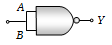(a) NAND gate
(b) OR gate
(c) AND gate
(d) NOR gate

Concept Questions :-

Logic gates
High Yielding Test Series + Question Bank - NEET 2020

Difficulty Level:

Symbol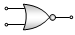(a) NAND gate                  (b) NOR gate
(c) NOT gate                     (d) XNOR gate

Concept Questions :-

Logic gates
High Yielding Test Series + Question Bank - NEET 2020

Difficulty Level:

To get an output 1 from the circuit shown in the figure, the input must be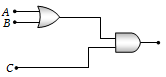(a) A = 0, B = 1, C = 0
(b) A = 1, B = 0, C = 0
(c) A = 1, B = 0, C = 1
(d) A = 1, B = 1, C = 0

Concept Questions :-

Logic gates
High Yielding Test Series + Question Bank - NEET 2020

Difficulty Level:

The combination of the gates shown in the figure below produces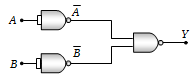(a) NOR gate
(b) OR gate
(c) AND gate
(d) XOR gate

Concept Questions :-

Logic gates
High Yielding Test Series + Question Bank - NEET 2020

Difficulty Level:

Which logic gate is represented by the following combination of logic gates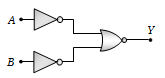(a) OR             (b) NAND
(c) AND           (d) NOR

Concept Questions :-

Logic gates
High Yielding Test Series + Question Bank - NEET 2020

Difficulty Level:

The output of OR gate is 1

(a) If both inputs are zero

(b) If either or both inputs are 1

(c) Only if both input are 1

(d) If either input is zero

Concept Questions :-

Logic gates
High Yielding Test Series + Question Bank - NEET 2020

Difficulty Level:

Which gates is represented by this figure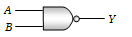(a) NAND gate
(b) AND gate
(c) NOT gate
(d) OR gate

Concept Questions :-

Logic gates
High Yielding Test Series + Question Bank - NEET 2020

Difficulty Level:

Which of the following logic gate is an universal gate?

1. OR

2. NOT

3. AND

4. NOR

Concept Questions :-

Logic gates
High Yielding Test Series + Question Bank - NEET 2020

Difficulty Level:

In a crystal, the atoms are located at the position of

(a) Maximum potential energy

(b) Minimum potential energy

(c) Zero potential energy

(d) Infinite potential energy

Concept Questions :-

Types of semiconductors